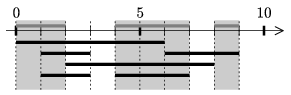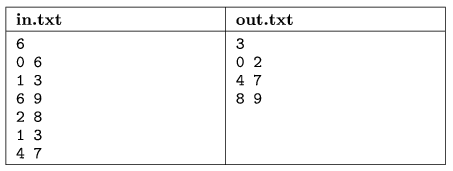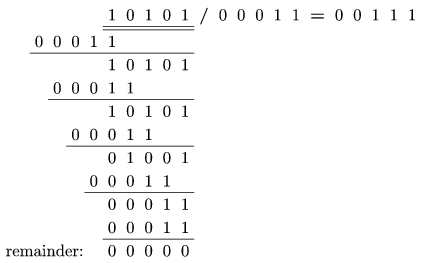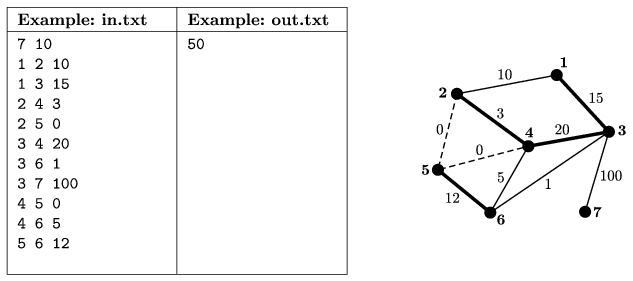Mathematical and Physical Journal
for High Schools
Issued by the MATFUND Foundation
 Already signed up? New to KöMaL?

# KöMaL Problems in Informatics, January 2008

Show/hide problems of signs:## Problems with sign 'I'

Deadline expired on February 15, 2008.

I. 175. We are given N closed intervals on the number line. Determine all intervals on the line which are contained in an odd number of the given ones. The endpoints of the intervals do not need to be considered. Your solution should consist of the minimal number of intervals, that is, the intervals should be disjoint and neighbouring ones should be merged.

The name of the input and output files are given as the first and second command line arguments of your program (e.g. i175 in.txt out.txt). The first line of the input file is the number of intervals (0N7000), then each of the next N lines contains two integers X and Y (separated by a space) describing the endpoints of an interval (). The first line of the output file should contain the number of intervals M, then each of the next M lines should describe these intervals (with endpoints in increasing order) in the same format as the input.The source code of your program (i175.pas, i175.cpp, ...) together with a short documentation (i175.txt, i175.pdf, ...) should be submitted, also containing a short description of your solution and the name of the developer environment to use.

(10 pont)

solution (in Hungarian), statistics

I. 176. The following problem was proposed at the International Mathematical Olympiad in 1988.

Consider two coplanar circles of different radii with the same center O. Let P be a fixed point on the smaller circle and B a variable point on the larger circle. The line BP meets the larger circle again at C. The perpendicular l to BP at P meets the smaller circle again at A (if l is tangent to the circle at P, then A=P). Find the locus of the midpoint of AB.

Solve this problem using the GeoGebra program (freely downloadable from the Internet). Apply the notations above. Make an animation consisting of at least 20 frames of size 400×400 pixels. The locus of the midpoint should be visible while B runs on the rim of the larger circle.

The GeoGebra file i176.ggb should be submitted together with an animated GIF file i176.gif.

(10 pont)

solution (in Hungarian), statistics

I. 177. Make a spreadsheet to carry out long division using binary numbers.

In the first row of the sheet, at a given location, we should be able to enter two binary numbers with at most five digits. Each digit is entered into a different cell. Then your sheet should display all the partial results of the long division of these two numbers as well as the quotient and the remainder.The spreadsheet (i177.xls, i177.ods, ...) together with a short documentation (i177.txt, i177.pdf, ...) should be submitted, also containing a short description of your solution and the name and version number of the spreadsheet application.

(10 pont)

solution (in Hungarian), statistics## Problems with sign 'S'

Deadline expired on February 15, 2008.

S. 32. One can get to every showplace in a national park on bikeways as well. Some bikeways however are damaged by the winter weather. The damages have been estimated and a reconstruction value has been assigned to every bikeway expressing how important it would be to repair that bikeway.

The most important bikeways have to be reconstructed before the summer. It is required that during the reconstruction visitors should still be able to get to every showplace on bikeways. There is no limit on how many bikeways can be reconstructed at the same time, but reconstructing all of them is possible only during the whole spring.

Write a program to determine at most how many bikeways can be repaired during spring. According to this plan, you should also determine the maximum of the sums of reconstruction values of these bikeways.

The input file contains the description of the bikeways and the result is written to the output file. The names of these files are given as the first and second command line arguments of your program (e.g. s32 in.txt out.txt).

The first line of the input contains the number of showplaces N (at most 100) and the number of bikeways M (at most 500) connecting them, separated by spaces. Showplaces are denoted by integers. Then each of the following M lines contains three positive integers separated by a space in a form A B R, representing that this bikeway connects (in both directions) showplaces A and B, and has reconstruction value R>0 (an integer between 1 and 1000). (If R=0, then currently no reconstruction is necessary on that bikeway.)

The output of your program is a single number giving the sum of reconstruction values of the bikeways if an optimal strategy is chosen.The source code of your program (s32.pas, s32.cpp, ...) together with a short documentation (s32.txt, s32.pdf, ...) should be submitted, also containing a short description of your solution and the name of the developer environment to use.

(10 pont)

solution (in Hungarian), statistics

\$Var(Body)